Bézier curves in mugen (Read 8291 times)

Started by Bea, October 02, 2005, 08:15:52 pm

Bea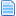Bézier curves in mugen
#1  October 02, 2005, 08:15:52 pm
••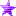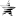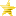• MUGEN Grandma
The idea:
- To implement a basic bézigon (path made of two or more bezier curves) using mugen script language.

Edit due to Val suggestion:

a bezier code is a smooth curve calculated from at least three points, using this code you can make your projectile fly smooth curves from position a to position b (good for homing projectiles etc).

The code below will generate two quadratics bézier curves with random control points starting at player position and ending out of screen. The child helper will smoothly follow the bézier curves creating a quite nice move. :P

The sources:
- http://www.gamedev.net/reference/articles/article888.asp
- http://www.gamedev.net/reference/articles/article1584.asp
- http://en.wikipedia.org/wiki/Bernstein_polynomial
- http://en.wikipedia.org/wiki/Bezier_curve

The result:

Quote
;---------------------------------------------------------------------------
; Bézier control points helper
[Statedef 3510]
type    = S
movetype= A
physics = N
velset = 0,0
ctrl = 0
anim = 9999
SprPriority = 2

[State 3510, Generate Point 0]
Type = Null
trigger1 = fvar(2) := 0
persistent = 0

[State 3510, Generate Point 0]
Type = Null
trigger1 = fvar(3) := pos y
persistent = 0

[State 3510, Generate Control points]
Type = Null
trigger1 = (1) || fvar(4) := 160 * random/1000.0 * facing; * ifelse(random < 500, 1, -1)
trigger1 = (1) || fvar(5) := -15 -60 * random/1000.0
trigger1 = (1) || fvar(6) := 320 * random/1000.0 * facing; * ifelse(random < 500, 1, -1)
trigger1 = (1) || fvar(7) := -10 -60 * random/1000.0 + 120 * ifelse(random < 500, 0, 1)
trigger1 = (1) || fvar(8) := 320 * random/1000.0 * facing; * ifelse(random < 500, 1, -1)
trigger1 = (1) || fvar(9) := -10 -60 * random/1000.0
trigger1 = (1) || fvar(10) := 320 * random/1000.0 * facing; * ifelse(random < 500, 1, -1)
trigger1 = (1) || fvar(11) := -10 -60 * random/1000.0
trigger1 = (1) || fvar(12) := 320 * random/1000.0 * facing; * ifelse(random < 500, 1, -1)
trigger1 = (1) || fvar(13) := -15 -60 * random/1000.0 + 120 * ifelse(random < 500, 0, 1)
trigger1 = (1) || fvar(14) := (320 + 80 * random/1000.0) * facing; * ifelse(random < 500, 1, -1)
trigger1 = (1) || fvar(15) := -240 * random/1000.0
persistent = 0

;Spawn the helpers
[State 3510, Helpers]
Type = Helper
trigger1 = time = 0
stateno = 3512
ownpal = 1
id = 3510
pos = 0,0

[State 3510, DestroySelf]
Type = DestroySelf
trigger1 = numhelper(3510) = 0

;---------------------------------------------------------------------------
; Bézier points
[Statedef 3512]
type    = S
movetype= A
physics = N
velset = 0,0
ctrl = 0
anim = 3310
SprPriority = 2

Type = AssertSpecial
trigger1 = 1

[State 3512, VarSet]
Type = VarSet
trigger1 = var(1) = 0
var(1) = parent, var(0)

[State 3512, Control how many steps I had taken]
trigger1=  fvar(11) >= 1.0
v = 4
value = 1

[State 3512, A]
Type = VarSet
trigger1 = var(0) = 0
trigger2 = fvar(11) >= 1.0
fvar(10) = 1.0
ignorehitpause = 1

[State 3512, A]
Type = VarSet
trigger1 = var(0) = 0
trigger2 = fvar(11) >= 1.0
fvar(11) = 0.0
ignorehitpause = 1

[State 3512, Generate Control points]
Type = Null
trigger1 = fvar(11) = 0.0
trigger1 = (1) || fvar(2) := ifelse(var(4) = 0, parent, fvar(2), fvar(8))
trigger1 = (1) || fvar(3) := ifelse(var(4) = 0, parent, fvar(3), fvar(9))
trigger1 = (1) || fvar(4) := parent, fvar(4 + var(4) * 6)
trigger1 = (1) || fvar(5) := parent, fvar(5 + var(4) * 6)
trigger1 = (1) || fvar(6) := parent, fvar(6 + var(4) * 6)
trigger1 = (1) || fvar(7) := parent, fvar(7 + var(4) * 6)
trigger1 = (1) || fvar(8) := parent, fvar(8 + var(4) * 6)
trigger1 = (1) || fvar(9) := parent, fvar(9 + var(4) * 6)

[State 3512, Generate X my offset]
Type = varset
trigger1 = 1
fvar(0)= fvar(2)*fvar(10)*fvar(10)*fvar(10)+ 3 * fvar(4)*fvar(10)*fvar(10)*fvar(11)
ignorehitpause = 1

[State 3512, Generate X my offset]
Type = varset
trigger1 = 1
fvar(0) = fvar(0)+ 3 * fvar(6)*fvar(10)*fvar(11)*fvar(11) + fvar(8)*fvar(11)*fvar(11)*fvar(11)
ignorehitpause = 1

[State 3512, Generate Y my offset]
Type = varset
trigger1 = 1
fvar(1)=fvar(3)*fvar(10)*fvar(10)*fvar(10)+ 3 * fvar(5)*fvar(10)*fvar(10)*fvar(11)
ignorehitpause = 1

[State 3512, Generate Y my offset]
Type = varset
trigger1 = 1
fvar(1) = fvar(1)+ 3 * fvar(7)*fvar(10)*fvar(11)*fvar(11)+fvar(9)*fvar(11)*fvar(11)*fvar(11)
ignorehitpause = 1

[State -3, M]
Type = VarSet
trigger1 = Pos X - (fvar(0) + (parent, pos x)) != 0
fvar(12) = -((Pos Y - fvar(1))/(Pos X - (fvar(0) + (parent, pos x)))* facing)

[State -3, M] ;There can be no triangle if both points share the same X cord
Type = VarSet
trigger1 = Pos X - (fvar(0) + (parent, pos x)) = 0
fvar(12) = 0

[State -3, Angle]
Type = AngleDraw
trigger1 = 1
value = Atan(fvar(12)) * (180.0/Pi) + 180 * ifelse(facing=1,(Pos X - (fvar(0) + (parent, pos x)) > 0),(Pos X - (fvar(0) + (parent, pos x)) < 0))

[State 3512, PosSet]
Type = PosSet
trigger1 = 1
x = fvar(0) + (parent, pos x)
y = fvar(1)
ignorehitpause = 1

[State 3512, A]
trigger1 = 1
fv = 10
value = -0.02
ignorehitpause = 1

[State 3512, A]
trigger1 = 1
fv = 11
value = 0.02
ignorehitpause = 1

[State 3512, Pause]
Type = Trans
trigger1 = 1
ignorehitpause = 1

[State 3512, DestroySelf]
Type = DestroySelf
trigger1 = var(4) = 2
ignorehitpause = 1

How it works:
- The mother helper creates several control points, saving them in fvar(4) and onward
- Each node iteration requires 4 points (we're using quadratic bézier curves here). For each node bigger than 1, the start point is last node end point
- For a path with N nodes, the mother helper needs to generate 4 * N points. In our example the starting point fvar(2), fvar(3) is 0,0.
- In the child helper, var(4) represents the number of nodes our path will have
- Each control point is made of a set of fvars. Fvars with even numbered indexes represents the X component of the point. Fvars with odd numbered indexes represents the Y component of the point.
- The X component of the control point must be adjusted by facing to guarantee that the curve will follow the right direction.
- In the child helper, fvar(10) and fvar(11) means the amount of the curve already done in parts of 1.0. They're increased and decrease by a fixed amount which must be a fraction of 1.0. The size of this increment determines how many points it will take to fully iterate a node. To find out the increment, divide 1.0 per the number of points you want to draw in each curve. In our example here we have 50 points (1.0/50 = 0.02)
- In the child helper, fvar(10) is always incremented and fvar(11) is always decremented
- The child helper destroys itself when he finishs the whole path, saving in var(4) the number of nodes already completed.
- The given code computes the angle between the current point and the next one and apply it to the child helper.

That's it. If you got any other doubt, ask.

Kisses.
Princess Adora: "My friend saw She-Ra take her dress off in the shower. She said she has an 8 pack. She said She-Ra is shredded."

SF2NES is dead. Long live SF2NES.
Last Edit: August 21, 2007, 05:37:12 pm by Bia

Winane#2  February 04, 2007, 11:21:45 pm
••• Tends to lose track of things a lot. :/
Mind fixing all the characters above that got screwed up during the board's UTF-8 conversion a few months ago?

Also, "bellow" != "below".
And the grammar in this sentence needs correcting:
In our example where have 50 points (1.0/50 = 0.02)

(If you want me to, I'll point out additional grammar and spelling errors to correct.  These here were just the ones that seemed most significant.)
Still quite busy.

(Yes, I intend to deal with that stuff eventually, but kinda can't just yet, sorry. :/ )

Bea#3  February 05, 2007, 01:51:02 am
••• MUGEN Grandma
Done fixing the characters and the grammar.

Feel free to point the other ones so I can fix them.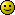Princess Adora: "My friend saw She-Ra take her dress off in the shower. She said she has an 8 pack. She said She-Ra is shredded."

SF2NES is dead. Long live SF2NES.
Last Edit: February 05, 2007, 01:53:49 am by Bia

eddy515#4  February 20, 2007, 09:47:22 pm
••• Get Ur mind out the gutter
what does this do exactly?
improve AI aiming?

brezier curve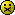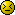<------hey! this site changed my sig!

Bea#5  February 21, 2007, 02:21:49 am
••• MUGEN Grandma
It makes a helper follow a smooth path using key points to calculate the missing points...

To put it simple, it calculate the points of a bÃ©zier curve.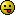Princess Adora: "My friend saw She-Ra take her dress off in the shower. She said she has an 8 pack. She said She-Ra is shredded."

SF2NES is dead. Long live SF2NES.

Cybaster#6  February 21, 2007, 03:50:27 pm
••• Limited time to use Infinite power !
@ eddy : the link provided by Bia here mainly tells you all you need about what BÃ©zier curves are.

Why do new cars have so smooth curves ? Just thank BÃ©zier. 8)

eddy515Re: Bézier curves in mugen
#7  February 22, 2007, 10:39:20 pm
••• Get Ur mind out the gutter
Quote
Cybaster: the link provided by "Bia"(hehe) here mainly tells you all you need about what Bézier curves are
(what the!)......
...(can't beleive I have 2 read)...
......
OOOOOooooh.
I feel all college-educated (hasn't bn 2 college yet)
let me see if I get this. (ahem)

"This code enables helpers to change their mind(of direction) following U?"
^^^^(or gives the appearance of, grammer grammer)
(Olive won't have 2 retrace Popeye's movements)

This cod 4 the prog or future chars?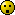WoW (so much work)<------hey! this site changed my sig!
Last Edit: February 22, 2007, 11:36:58 pm by eddy515

greetlockRe: Bézier curves in mugen
#8  October 17, 2007, 09:08:08 pm
••damn, either you guys are geniuses from harvard, or I missed out on a couple of things during high school and college, because this is all like trying to read Japanese for me (no offense to Japanese). This just makes me want to go back to college and study all there is about Arithmetic or however you want to call it. I feel left out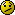, lost ???, deceived, misguided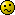, time wasted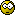, stupid, retarded, and fat... Although the last one is truly my fault, LOL...

I would like to learn all there is about this Var, and Fvar, and cos, and sin, and ifelse, and all the weird stuff in mugen, that I could read it and understand exactly what they mean, cuz I really don't understand siht...

When I read all this, I feel like you guys are doing to me what that girl in eddy515's avatar is doing to the guy...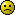........

ElixRe: Bézier curves in mugen
#9  October 18, 2007, 05:21:37 pm
••• "Moyashi tsuku shite yaru..."
I would like to learn all there is about this Var, and Fvar, and cos, and sin, and ifelse, and all the weird stuff in mugen, that I could read it and understand exactly what they mean, cuz I really don't understand siht...

When I read all this, I feel like you guys are doing to me what that girl in eddy515's avatar is doing to the guy...Be patient and learn. I was almost like you a year ago